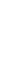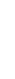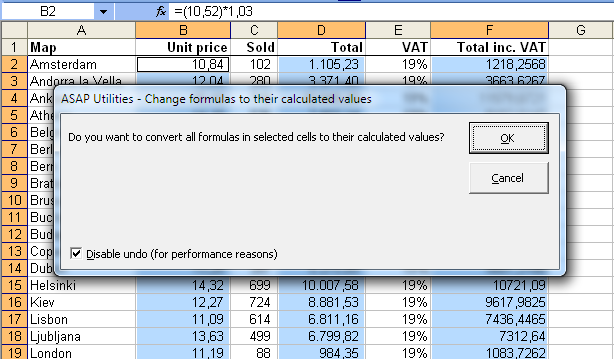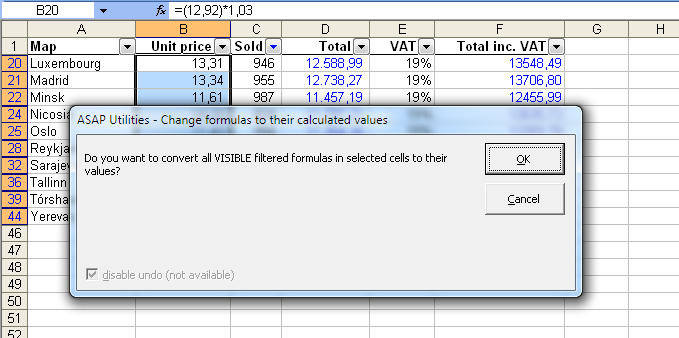# ASAP Utilities, description of our Excel toolsEnglish (us) / Nederlands / Deutsch / Español / Français / Português do Brasil / Italiano / Русский / 中文(简体) / 日本語

## Formulas » Change formulas to their calculated values

This utility replaces the formulas in the selected cells with their calculated values.

The utility works the same as Excel's 'Copy » Paste special » Paste values' but in one step and it also works with multiple selections at once.
Normally the utility changes the formulas to their calculated values in both visible and hidden cells. If you have selected cells in a filtered list only the visible cells are changed.

As an example, a cell with the formula '=A2*(C19/12)' which has '124' as the calculated result will be replaced with the value 124.
This can be useful, for example if you want to speed up the calculation of your workbook or if you don't want someone to see the underlying formulas you used if you give the workbook to somebody else.

By default you can start this utility with the shortcut Ctrl+Alt+P.

## Screenshots

Example screenshot: 1 Change formulas to their calculated valuesExample screenshot: 2 Formulas to values in a filtered list is something that you normally can't do in Excel## Starting this utility

In the Excel menu choose: ASAP Utilities » Formulas » Change formulas to their calculated values